# AP SSC 10th Class Maths Solutions Chapter 3 Polynomials InText Questions

## AP State Syllabus SSC 10th Class Maths Solutions 3rd Lesson Polynomials InText Questions

AP State Board Syllabus AP SSC 10th Class Maths Textbook Solutions Chapter 3 Polynomials InText Questions and Answers.

### 10th Class Maths 3rd Lesson Polynomials InText Questions and Answers

Do these

Question 1.
State which of the following are polynomials and which are not? Give reasons.   (Page No. 48)
(i) 2x3
(ii) $$\frac{1}{x-1}$$
(iii) 4z2 + $$\frac{1}{7}$$
(iv) m2 – √2 m + 2
(v) p-2 + 1
i) 2x3 is a polynomial.
ii) $$\frac{1}{x-1}$$ is not a polynomial because its power is negative integer exponent.
iii) 4z2 + $$\frac{1}{7}$$ is a polynomial.
iv) m2 – √2 m + 2 is a polynomial.
v) p-2 + 1 is not a polynomial because its power is negative integer exponent.Question 2.
p(x) = x2 – 5x – 6, find the values of p(l), p(2), p(3), p(0), p(-l), p(-2), p(-3).    (Page No. 49)
Given polynomial p(x) = x2 – 5x – 6
p(1) = (1)2 – 5(1) – 6 = 1 – 5 – 6 = – 10
p(2) = (2)2 – 5(2) – 6 = 4 – 10 – 6 = -12
p(3) = 32 – 5(3) – 6 = 9 – 15 – 6 = – 12
p(0) = 02 – 5(0) – 6 = – 6
p(-1) = (-1)2 – 5(-1) – 6 = 1 + 5 – 6 = 0
p(-2) = (-2)2 – 5(-2) – 6 = 4 + 10 – 6 = 8
p(-3) = (-3)2 – 5(-3) – 6 = 9 + 15 – 6 = 18

Question 3.
p(m) = m2 – 3m + 1, find the values of p(1)and p(-1).    (Page No. 49)
Given polynomial p(m) = m2 – 3m + 1
p(1) = (1)2 – 3(1) + 1 = 1 – 3 + 1 = 2 – 3 = – 1
p(-1) = (-1)2 – 3(-1) + 1 = 1 + 3 + 1 = 5

Question 4.
Let p(x) = x2 – 4x + 3. Find the values of p(0), p(l), p(2), p(3) and obtain zeroes of the polynomial p(x).   (Page No. 50)
Given polynomial p(x) = x2 – 4x + 3
p(0) = (0)2 – 4(0) + 3 = 3
p(1) = (1)2 – 4(1) + 3 = 1 – 4 + 3 = 0
p(2) = (2)2 – 4(2) + 3 = 4 – 8 + 3 = – 1
p(3) = (3)2 – 4(3) + 3 = 9 – 12 + 3 = 0
We see that p(1) and p(3) are zeroes of the polynomial p(x).Question 5.
Check whether -3 and 3 are the zeroes of the polynomial x2 – 9.    (Page No. 50)
Given polynomial p(x) = x2 – 9
Zero of the polynomial p(x) = 0
x2 – 9 = 0
⇒ x2 = 9
⇒ x = V9 = ± 3
∴ x = + 3, – 3
∴ Zeroes of the polynomial p(x) are – 3 and 3.

Try these

Question 1.
Write 3 different quadratic, cubic and 2 linear polynomials with different number of terms. (Page No. 48)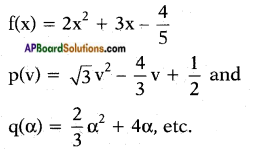Cubic polynomials :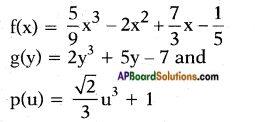Linear polynomials :
f(t) = √2 t + 5
g(u) = $$\frac{2}{3}$$ u – $$\frac{5}{2}$$ and
q(y) = 3y
Yes, we can write polynomials of any degree.

Question 2.
Write a quadratic polynomial and a cubic polynomial in variable x in the general form. (Page No. 49)
General form of a quadratic polynomial having variable ‘x’ is
f(x) = ax3 + bx2 + c, a ≠ 0
General form of a cubic polynomial having variable ‘x’ is
f(x) = ax3 + bx2 + cx + d, a ≠ 0Question 3.
Write a general polynomial q(z) of degree n with coefficients that are b0…. bn. What are the conditions on b0…. bn. (Page No. 49)
q(z) = b0zn + b1zn-1 + b2zn-2 …….. + bn-1z + bn is a polynomial of n degree where b0, b1, b2,…… bn-1, bn are real coefficients and b0 ≠ 0.

Do this

Question 1.
Draw the graph of i) y = 2x + 5, ii) y = 2x – 5, iii) y = 2x and find the point of intersection on X – axis. Is the x-coordinates of these points also the zero of the polynomial?   (Page No. 52)
i) Given that y = 2x + 5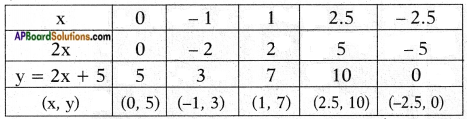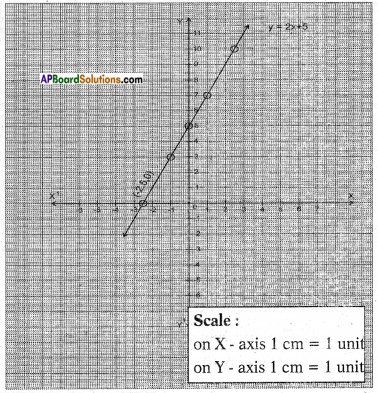Result: The graph y = 2x + 5 cuts the X – axis at the point (-2.5, 0).
Hence, the zeroes of the polynomial is -2.5.

ii) Given that y = 2x – 5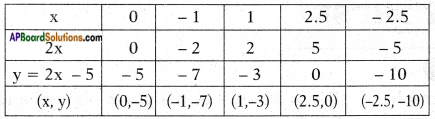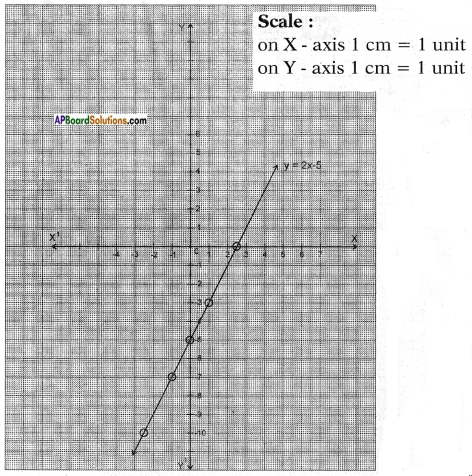Result: The graph of y = 2x – 5 cuts the X – axis at the point (2.5, 0).
The zeroes of the polynomial is 2.5 = $$\frac{5}{2}$$iii) Given that y = 2x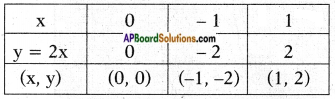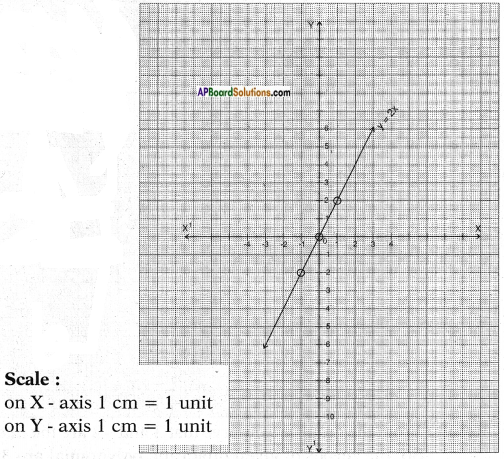Result: The graph passes through the origin.
So, the zeroes of the polynomial y = 2x is zero.

Try these

Question 1.
Draw the graphs of (i) y = x2 – x – 6 (ii) y = 6 – x – x2 and find zeroes in each case. What do you notice? (Page No. 53)
i) Given that y = x2 – x – 6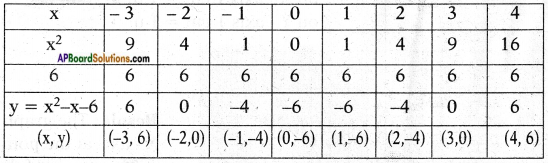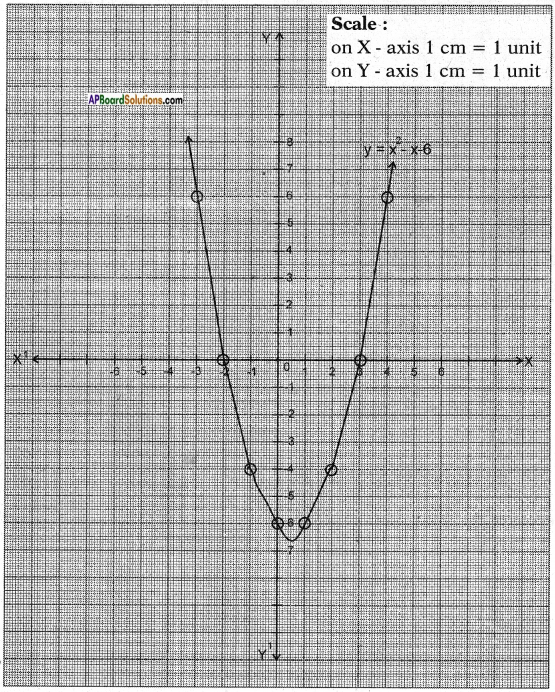Result: From the graph we observe that 3 and -2 are the intersecting points of X – axis.
So, the zeroes of given quadratic polynomial are 3 and -2.ii) Given that y = 6 – x – x2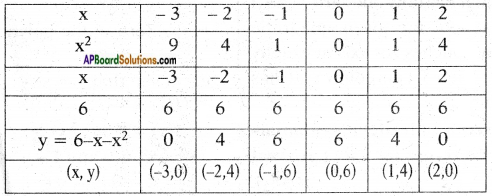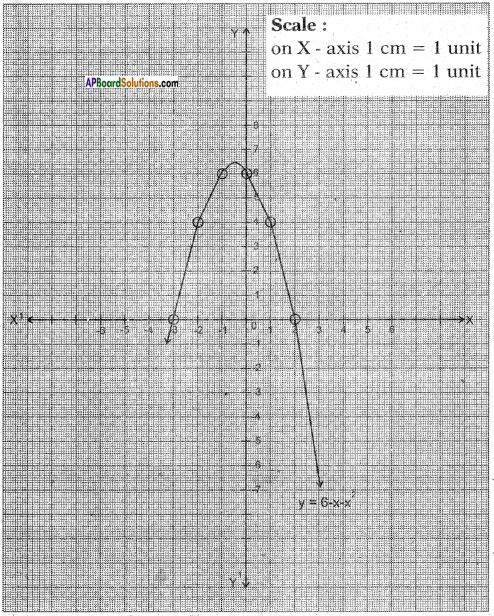Result: From the graph we observe that – 3 and 2 are the intersecting points ol X – axis.
So, the zeroes of given quadratic polynomial are – 3 and 2.

Question 2.
Write three quadratic polynomials that have 2 zeroes each.   (Page No. 55)
y = x2 – x – 2 having two zeroes, i.e., (2, 0) and (- 1, 0).
y = 3 – 2x – x2 having two zeroes i.e., (1,0) and (- 3, 0).
y = x2 – 3x – 4 having two zeroes i.e., (-1, 0) and (4, 0)Question 3.
Write one quadratic polynomial that has one zero. (Page No. 55)
Quadratic Polynomial y = x2 – 6x + 9 has only one zero i.e., 3.

Question 4.
How will you verify if a quadratic polynomial it has only one zero?  (Page No. 55)
If the graph of the given quadratic polynomial touches X – axis at exactly one point, then I can confirm it has only one zero.

Question 5.
Write three quadratic polynomials that have no zeroes for x that are real numbers.  (Page No. 55)
The quadratic polynomials y = 2x2 – 4x + 5 and y = – 3x2 + 2x – 1 and y = x2 – 2x + 4 have no zeroes.

Question 6.
Find the zeroes of cubic polynomials
(i) – x3
(ii) x2 – x3
(iii) x3 – 5x2 + 6x
without drawing the graph of the polynomial.  (Page No. 57)
i) Given polynomial is y = – x3
f(x) = -x3 ; f(x) = 0
x3 = 0
x = $$\sqrt{0}$$ = 0
∴ Zero of the polynomial f(x) is only one i.e., 0.ii) Given that y = x2 – x3
f(x) = x2 (1 – x)
f(x) = 0
⇒ x2 (1 – x) = 0
⇒ x2 = 0 and 1 – x = 0
⇒ x = 0 and x = 1
∴ The zeroes of the polynomial f(x) are two i.e., 0 and 1.

iii) Given that x3 – 5x2 + 6x Let f(x) = x3 – 5x2 + 6x
= x(x2 – 5x + 6)
= x(x2 – 2x – 3x + 6)
= x[x(x – 2) – 3(x – 2)]
= x(x – 2) (x – 3)
∴ The zeroes of the polynomial f(x) are x = 0 and x = 2 and x = 3

Do these

Question 1.
Find the zeroes of the quadratic polynomials given below. Find the sum and product of the zeroes and verify relationship to the coefficients of terms in the polynomial. (Page No. 62)
i) p(x) = x2 – x – 6
ii) p(x) = x2 – 4x + 3
iii) p(x) = x2 – 4
iv) p(x) = x2 + 2x + 1
i) Given polynomial p(x) = x2 – x – 6
We have x2 – x – 6 = x2 – 3x + 2x – 6
= x(x – 3) + 2(x – 3)
= (x – 3) (x + 2)
So, the value of x2 – x – 6 is zero when x – 3 = 0 or x + 2 = 0
i.e., x = 3 or x = -2
So, the zeroes of x2 – x – 6 are 3 and – 2.
∴ Sum of the zeroes = 3 – 2 = 1
= – $$\frac{\text { Coefficient of } x}{\text { Coefficient of } x^{2}}$$ = $$\frac{-(-1)}{1}$$ = 1
And product of the zeroes = 3 × (-2) = -6
= $$\frac{\text { Constant term }}{\text { Coefficient of } x^{2}}$$ = $$\frac{-6}{1}$$ = -6

ii) p(x) = x2 – 4x + 3
Given polynomial p(x) = x2 – 4x + 3
We have, x2 – 4x + 3 = x2 – 3x – x + 3
= x(x – 3) – 1 (x – 3)
= (x – 3) (x – 1)
So, the value of x2 – 4x + 3 is zero when x – 3 = 0 or x – 1 =0, i.e.,
when x = 3 or x = 1 So, the zeroes of x2 – 4x + 3 are 3 and 1
∴ Sum of the zeroes = 3 + 1 = 4
= – $$\frac{\text { Coefficient of } x}{\text { Coefficient of } x^{2}}$$ = $$\frac{-(-4)}{1}$$ = 4
And product of the zeroes = 3 × 1 = 3
= $$\frac{\text { Constant term }}{\text { Coefficient of } x^{2}}$$ = $$\frac{3}{1}$$ = 3iii) Given polynomial p(x) = x2 – 4
We have, x2 – 4 = (x – 2) (x + 2)
So, the value of x2 – 4 is zero
when x – 2 = 0 or x + 2 = 0
i.e., x = 2 or x = – 2
So the zeroes of x2 – 4 are 2 and – 2
∴ Sum of the zeroes = 2 + (- 2) = 0
= – $$\frac{\text { Coefficient of } x}{\text { Coefficient of } x^{2}}$$ = $$\frac{-0}{1}$$ = 0
And product of the zeroes = 2 × (-2) = -4
= $$\frac{\text { Constant term }}{\text { Coefficient of } x^{2}}$$ = $$\frac{-4}{1}$$ = -4

iv) Given polynomial p(x) = x2 + 2x + 1
We have x2 + 2x + 1 = x2 + x + x + 1
= x(x + 1) + l(x + 1)
= (x + 1) (x + 1)
So, the value of x2 + 2x + 1 is zero
when x + 1 = 0 (or) x + 1 = 0, i.e.,
when x = – 1 or – 1
o, the zeroes of x2 + 2x + 1 are – 1 and – 1.
∴ Sum of the zeroes = (-1) + (-1) = -2
= – $$\frac{\text { Coefficient of } x}{\text { Coefficient of } x^{2}}$$ = $$\frac{-2}{1}$$ = -2
And product of the zeroes = (-1) × (-1) = 1
= $$\frac{\text { Constant term }}{\text { Coefficient of } x^{2}}$$ = $$\frac{1}{1}$$ = 1

Question 2.
If α, β and γ are the zeroes of the given cubic polynomials, find the values as given in the table. (Page No. 66)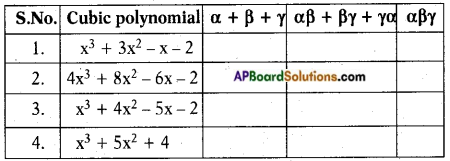l) Given polynomial is x3 + 3x2 – x – 2.
Comparing given polynomial with ax3 + bx2 + cx + d,
the values are a = 1, b = 3, c = -l, d = -2
α + β + γ = $$\frac{-b}{a}$$ = $$\frac{-3}{1}$$ = -3
αβ + βγ + γα = $$\frac{c}{a}$$ = $$\frac{-1}{1}$$ = -1
αβγ = $$\frac{-d}{a}$$ = $$\frac{-(-2)}{1}$$ = 22) Given polynomial is 4x3 + 8x2 – 6x – 2
Compare the polynomial with ax3 + bx2 + cx + d = 0
Then a = 4, b = 8, c = – 6 and d = – 2
α + β + γ = $$\frac{-b}{a}$$ = $$\frac{-8}{4}$$ = -2
αβ + βγ + γα = $$\frac{c}{a}$$ = $$\frac{-6}{4}$$ = $$\frac{-3}{2}$$
αβγ = $$\frac{-d}{a}$$ = $$\frac{-(-2)}{4}$$ = $$\frac{1}{2}$$

3) Given polynomial is x3 + 4x2 – 5x – 2
Compare the polynomial with ax3 + bx2 + cx + d = 0
Then a = 1, b = 4, c = – 5 and d = – 2
α + β + γ = $$\frac{-b}{a}$$ = $$\frac{-4}{1}$$ = -4
αβ + βγ + γα = $$\frac{c}{a}$$ = $$\frac{-5}{1}$$ = -5
αβγ = $$\frac{-d}{a}$$ = $$\frac{-(-2)}{1}$$ = 2

4) Given polynomial is x3 + 5x2 + 4
Compare the polynomial with ax3 + bx2 + cx + d = 0
Then a = 1, b = 5, c = 0 and d = 4
α + β + γ = $$\frac{-b}{a}$$ = $$\frac{-5}{1}$$ = -5
αβ + βγ + γα = $$\frac{c}{a}$$ = $$\frac{0}{1}$$ = 0
αβγ = $$\frac{-d}{a}$$ = $$\frac{-4}{1}$$ = -4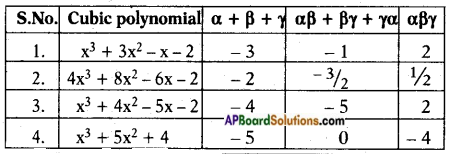Try this

Question 1.
i) Find a quadratic polynomial with zeroes -2 and $$\frac{1}{3}$$. (Page No. 64)
Let the quadratic polynomial be ax2 + bx + c, a ≠ 0 and its zeroes be α and β.
Here α = – 2 and β = $$\frac{1}{3}$$
Sum of the zeroes = α + β
= -2 + $$\frac{1}{3}$$ = $$\frac{-5}{3}$$
Product of the zeroes = αβ
= $$\frac{1}{3}$$ × (-2) = $$\frac{-2}{3}$$
∴ ax2 + bx + c is [x2 – (α + β)x + αβ]
= [x2 – $$\left(\frac{-5}{3}\right)$$x + $$\left(\frac{-2}{3}\right)$$]
the quadratic polynomial will be 3x2 + 5x – 2.ii) What is the quadratic polynomial whose sum of zeroes is $$\frac{-3}{2}$$ and the product of zeroes is -1.
Here α + β = $$\frac{-3}{2}$$ and αβ = -1
= x2 – $$\left(\frac{-3}{2}\right)$$x + (-1)
= x2 + $$\frac{3x}{2}$$ – 1
The other polynomials are (x2 + $$\frac{3x}{2}$$ – 1)